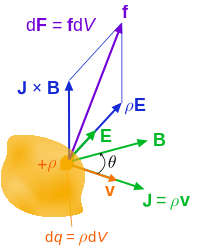# Maxwell stress tensor

The Maxwell stress tensor (named after James Clerk Maxwell) is a second rank tensor used in classical electromagnetism to represent the interaction between electromagnetic forces and mechanical momentum. In simple situations, such as a point charge moving freely in a homogeneous magnetic field, it is easy to calculate the forces on the charge from the Lorentz force law. When the situation becomes more complicated, this ordinary procedure can become impossibly difficult, with equations spanning multiple lines. It is therefore convenient to collect many of these terms in the Maxwell stress tensor, and to use tensor arithmetic to find the answer to the problem at hand.

In the relativistic formulation of electromagnetism, the Maxwell's tensor appears as a part of the electromagnetic stress–energy tensor which is the electromagnetic component of the total stress–energy tensor. The latter describes the density and flux of energy and momentum in spacetime.

## MotivationLorentz force (per unit 3-volume) f on a continuous charge distribution (charge density ρ) in motion. The 3-current density J corresponds to the motion of the charge element dq in volume element dV and varies throughout the continuum.

As outlined below, the electromagnetic force is written in terms of E and B, using vector calculus and Maxwell's equations symmetry in the terms containing E and B are sought for, and introducing the Maxwell stress tensor simplifies the result.

Maxwell's equations in SI units in vacuum
(for reference)
Name Differential form
Gauss's law (in vacuum)Gauss's law for magnetismAmpère's circuital law (in vacuum)
(with Maxwell's correction)1. Starting with the Lorentz force lawthe force per unit volume is2. Next, ρ and J can be replaced by the fields E and B, using Gauss's law and Ampère's circuital law:3. The time derivative can be rewritten to something that can be interpreted physically, namely the Poynting vector. Using the product rule and Faraday's law of induction givesand we can now rewrite f asthen collecting terms with E and B gives4. A term seems to be "missing" from the symmetry in E and B, which can be achieved by inserting (∇ • B)B because of Gauss' law for magnetism:Eliminating the curls (which are fairly complicated to calculate), using the vector calculus identity5. This expression contains every aspect of electromagnetism and momentum and is relatively easy to compute. It can be written more compactly by introducing the Maxwell stress tensor,and notice that all but the last term of f can be written as the divergence of the above, giving:,

As in the Poynting's theorem, the second term in the left side of above equation can be interpreted as time derivative of EM field's momentum density and this way, the above equation will be the law of conservation of momentum in classical electrodynamics.

where we have finally introduced the Poynting vector,in the above relation for conservation of momentum,is the momentum flux density and plays a role similar toin Poynting's theorem.

## Equation

In physics, the Maxwell stress tensor is the stress tensor of an electromagnetic field. As derived above in SI units, it is given by:,

where ε0 is the electric constant and μ0 is the magnetic constant, E is the electric field, B is the magnetic field and δij is Kronecker's delta. In Gaussian cgs unit, it is given by:,

where H is the magnetizing field.

An alternative way of expressing this tensor is:where ⊗ is the dyadic product, and the last tensor is the unit dyad::The element ij of the Maxwell stress tensor has units of momentum per unit of area times time and gives the flux of momentum parallel to the ith axis crossing a surface normal to the jth axis (in the negative direction) per unit of time.

These units can also be seen as units of force per unit of area (negative pressure), and the ij element of the tensor can also be interpreted as the force parallel to the ith axis suffered by a surface normal to the jth axis per unit of area. Indeed, the diagonal elements give the tension (pulling) acting on a differential area element normal to the corresponding axis. Unlike forces due to the pressure of an ideal gas, an area element in the electromagnetic field also feels a force in a direction that is not normal to the element. This shear is given by the off-diagonal elements of the stress tensor.

## Magnetism only

If the field is only magnetic (which is largely true in motors, for instance), some of the terms drop out, and the equation in SI units becomes:For cylindrical objects, such as the rotor of a motor, this is further simplified to:where r is the shear in the radial (outward from the cylinder) direction, and t is the shear in the tangential (around the cylinder) direction. It is the tangential force which spins the motor. Br is the flux density in the radial direction, and Bt is the flux density in the tangential direction.

## Eigenvalue

The eigenvalues of the Maxwell stress tensor are given by:These eigenvalues are easily obtained by iteratively applying the Matrix Determinant Lemma, in conjunction with the Sherman-Morrison Formula.

Noting that the characteristic equation matrix,, can be written aswherewe setApplying the Matrix Determinant Lemma once, this gives usApplying it again yields,From the last multiplicand on the RHS, we immediately see thatis one of the eigenvalues.

To find the inverse of, we use the Sherman-Morrison formula:Factoring out aterm in the determinant, we are left with finding the zeros of the rational function:Thus, once we solvewe obtain the other two eigenvalues.